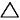# what mass of Pb^2+ ion is left in solution when 50 mL of 0.20 M Pb(NO3)2 is added to 50 mL of 1.5 NaCl? given k sp of PbCl2 is 1.7 x 10^-4

10 years ago

Dear Nancy,

1. Calculate the heat released, in J, by the precipitation reaction:

q = mass x specific heat capacity x change in temperature
q = [mPb(NO3)2(aq) + mKI(aq)] x cg x (Tf - Ti
q = [50 + 30] x 4.184 x (22.2 - 19.6) = 870.27 J

2. Calculate the moles of reactant specified, n[Pb(NO3)2(aq)]:

n = M x V
n[Pb(NO3)2(aq)] = 0.20 x 50 x 10-3 = 0.010 mol

3. Calculate the heat of precipitation,H, in kJ/mol of Pb(NO3)2(aq):H = -q/1000 ÷ nH = -0.870/1000 ÷ 0.010 = -87 kJ mol-1H is negative because the reaction is exothermic.

Cracking IIT just got more exciting,It s not just all about getting assistance from IITians, alongside Target Achievement and Rewards play an important role. ASKIITIANS has it all for you, wherein you get assistance only from IITians for your preparation and win by answering queries in the discussion forums.